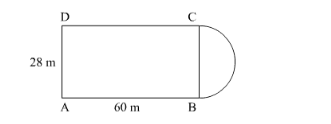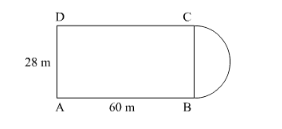# A plot is in the form of a rectangle ABCD having semi-circle on BCQuestion:

A plot is in the form of a rectangle ABCD having semi-circle on BC as shown in the following figure. If AB = 60 m and BC = 28 m, find the area of the plot.Solution:

It is given that a plot is in form of rectangle ABCD having a semicircle on BC.

$\mathrm{AB}=60 \mathrm{~m}$

$\mathrm{BC}=28 \mathrm{~m}$Since BC is the diameter of semicircle. Then, radius of semicircle is

$r=\frac{28}{2} \mathrm{~m}$

$=14 \mathrm{~m}$

Area of semicircle $=\frac{1}{2} \times \pi r^{2}$

$=\frac{1}{2} \times \frac{22}{7} \times 14 \times 14$

$=308 \mathrm{~m}^{2}$

Area of rectangle $\mathrm{ABCD}=l \times b$

$=60 \times 28$

$=1680 \mathrm{~m}^{2}$

Now,

Area of plot = Area of rectangle + Area of semicircle

$=1680+308 \mathrm{~m}^{2}$

$=1988 \mathrm{~m}^{2}$